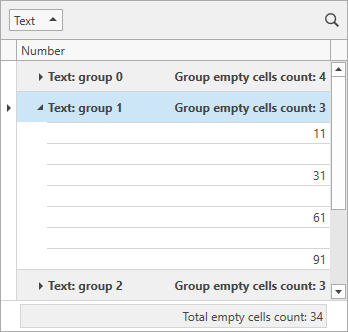# GridControl.CustomSummary Event

Allows you to calculate a custom summary.

Namespace: DevExpress.Xpf.Grid

Assembly: DevExpress.Xpf.Grid.v22.2.dll

NuGet Package: DevExpress.Wpf.Grid.Core

## Declaration

``public event CustomSummaryEventHandler CustomSummary``

## Event Data

The CustomSummary event's data class is CustomSummaryEventArgs. The following properties provide information specific to this event:

Property Description
FieldValue Gets the processed field value.
GroupLevel Gets the nested level of the group whose summary value is being calculated.
GroupRowHandle Gets a value identifying the group row whose child data rows are involved in summary calculation.
IsGroupSummary Gets whether a group summary value is being calculated.
IsTotalSummary Gets whether a total summary value is being calculated.
Item Gets a summary item whose value is being calculated.
Mode Specifies how summaries are calculated - against all rows or for the selected rows.
Row Gets the currently processed row.
RowHandle Gets the handle of the processed row.
SummaryProcess Gets a value indicating calculation stage.
TotalValue Gets or sets the total summary value.
TotalValueReady Gets or sets whether the Calculation stage of the custom summary calculation process should be skipped.

The event data class exposes the following methods:

Method Description
GetGroupSummary(Int32, Object) Returns the value of the specified group summary for the specified group row.
GetValue(String) Returns the value in the specified field

## Remarks

Total summaries and group summaries have predefined aggregate functions (COUNT, MAX, MIN, SUM, and AVG). To calculate custom summaries, handle the CustomSummary event. This event allows you to implement custom aggregate functions or use a custom algorithm to calculate summary values.

If the GridControl.View property is set to TreeListView, use the TreeListView.CustomSummary event.

Note

The CustomSummary event does not work in Server Mode.

If you want to maintain a clean MVVM pattern and specify custom summaries in a View Model, create a command and bind it to the CustomSummaryCommand property.

## Example

The following example demonstrates how to use custom summaries to count the total number of empty cells in the specified grid column:``````<dxg:GridControl x:Name="grid"
CustomSummary="OnCustomSummary">
<dxg:GridControl.Columns>
<dxg:GridColumn FieldName="Text" GroupIndex="0" />
<dxg:GridColumn FieldName="Number" />
</dxg:GridControl.Columns>
<dxg:GridControl.View>
<dxg:TableView x:Name="view"
AutoWidth="True"
TotalSummaryPosition="Bottom" />
</dxg:GridControl.View>
<dxg:GridControl.TotalSummary>
<dxg:GridSummaryItem DisplayFormat="Total empty cells count: {0}"
FieldName="Number"
SummaryType="Custom" />
</dxg:GridControl.TotalSummary>
<dxg:GridControl.GroupSummary>
<dxg:GridSummaryItem DisplayFormat="Group empty cells count: {0}"
FieldName="Number"
SummaryType="Custom" />
</dxg:GridControl.GroupSummary>
</dxg:GridControl>
``````
``````void OnCustomSummary(object sender, CustomSummaryEventArgs e) {
if(((GridSummaryItem)e.Item).FieldName != "Number")
return;
if(e.SummaryProcess == CustomSummaryProcess.Start) {
e.TotalValue = 0;
}
if(e.SummaryProcess == CustomSummaryProcess.Calculate) {
if(IsEmptyCell(e.FieldValue))
e.TotalValue = (int)e.TotalValue + 1;
}
}
bool IsEmptyCell(object fieldValue) {
return !((int?)fieldValue).HasValue;
}
``````

The following code snippets (auto-collected from DevExpress Examples) contain references to the CustomSummary event.

Note

The algorithm used to collect these code examples remains a work in progress. Accordingly, the links and snippets below may produce inaccurate results. If you encounter an issue with code examples below, please use the feedback form on this page to report the issue.